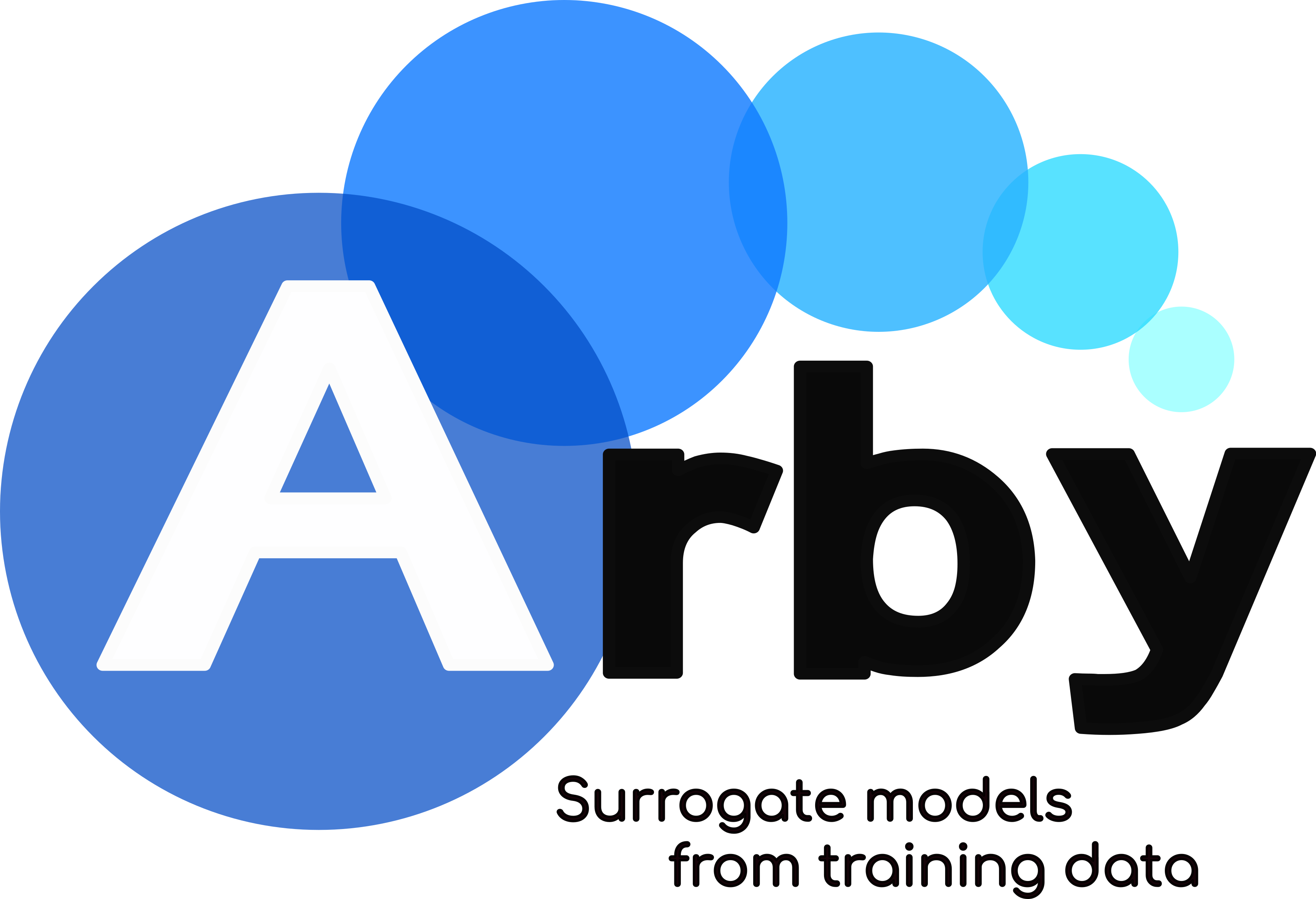# Arby’s documentation¶Arby is a fully data-driven Python module to construct surrogate models, reduced bases and empirical interpolants from training data.

This module implements a type of Reduced Order Modeling technique for reducing the computational complexity of mathematical models in numerical simulations.

# Quick Usage¶

Suppose we have a set of real functions parametrized by a real number $$\lambda$$. This set, the training set, represents an underlying parametrized model $$f_\lambda(x)$$ with continuous dependency in $$\lambda$$. Without complete knowledge about $$f_\lambda$$, we’d like to produce an accurate approximation only through the access to the training set.

With Arby we can build an accurate surrogate model to represent the training set. For simplicity, suppose a discretization of the parameter domain [par_min, par_max] with Ntrain samples indexing the training set

params = np.linspace(par_min, par_max, Ntrain)


and a discretization of the $$x$$ domain $$[a,b]$$ in Nsamples points

x_samples = np.linspace(a, b, Nsamples)


Next, we build a training set

training_set = [f(par, x_samples) for par in params]


that has shape (Ntrain, Nsamples).

Then we build the surrogate model with Arby by doing:

from arby import ReducedOrderModel as ROM
f_model = ROM(training_space=training_set,
physical_interval=x_samples,
parameter_interval=params)


With f_model we can get function samples for any parameter par in the interval [par_min, par_max] simply by calling it:

f_model_at_par = f_model.surrogate(par)
plt.plot(x_samples, model_at_par)
plt.show()